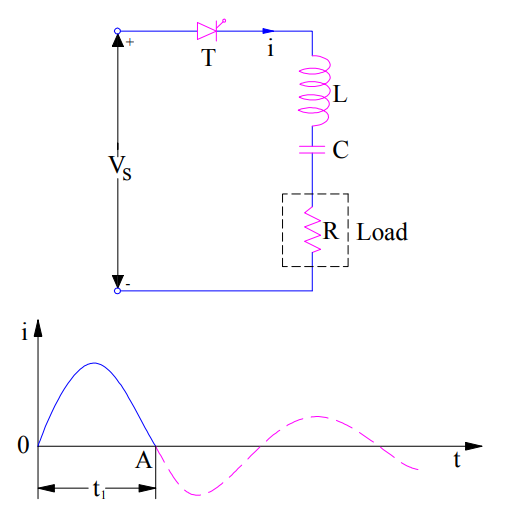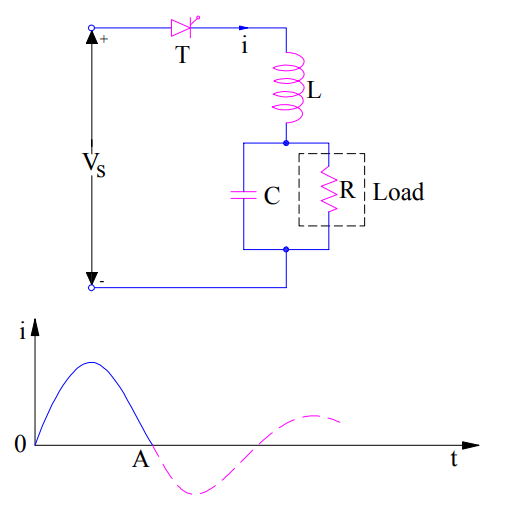# Load Commutation of SCR – Class A Commutation

Load commutation of SCR is a forced commutation technique in which SCR is forced to turn off. Turning off an SCR essentially requires its anode current to fall below holding current and a reversed biased voltage must be present across SCR for sufficient time so that SCR may regain its blocking state. Load Commutation of SCR is also known as Class-A commutation.

In Load Commutation, Capacitor C & Inductor L are used as commutating element. Commutating element L and C are connected in series with the load resistance if the value of load resistance is low. Circuit diagram for load commutation for low value of load resistance R is shown in figure below.If the value of load resistance is high, L is connected in series with resistor whereas C is connected across the load. This is shown in figure below.When the above circuit is energized from DC source, current waveform as shown in figure is obtained. As can be seen, the circuit current is under-damped in nature. The current is rising initially up to maximum and then begins to fall. When the current decays to zero (Capacitor is fully charges at instant A and hence will not allow further current flow) and tends to reverse. During the time when SCR conducts, voltage across SCR (VT) is zero. But as soon as current reaches zero and tend to reverse, a reverse voltage (since capacitor C is fully charged up to source voltage Vs, this capacitor voltage is applied across SCR) equal to the source voltage is applied across the terminals of SCR. Thus after time A, anode current of SCR is zero and it is reversed biased. The two conditions of SCR turn-off are met and hence SCR / thyristor is turned off on its own at instant A.

From the above discussion, it is quite clear that the main idea behind load commutation technique is to make an under-damped circuit. Since, thyristor is getting turned off on its own, this technique is also known as self-commutation or resonant commutation technique.

Class-A or Load commutation is prevalent in SCR circuit energized from a DC source like Series Inverter. The nature of the DC circuit should be such that when energized, the current should have a natural tendency to decay down to zero. Thus load commutation is only possible in DC circuit not in AC circuit.

This site uses Akismet to reduce spam. Learn how your comment data is processed.